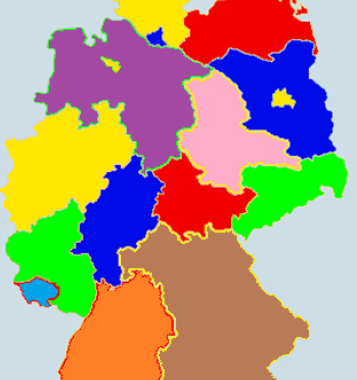## Map Coloring ProblemThis is a similar post as the one on Graph Coloring. However, this in this post I show an application of the graph coloring problem, allowing us to answer practical questions using graph theory. In this post we’ll first tackle a map coloring problem manually, then we’ll use a MiniZinc constraint programming script to validate the solution found.

## Graph Coloring ProblemA node coloring is valid or admissible if any two adjacent nodes do not have the same color. If a graph is colorable, there is a smallest number such that the graph is node colorable. This number is called the chromatic number of the graph and is usually denoted by . The problem to identify… Read more Graph Coloring Problem